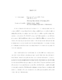## Estimating Common Odds Ratio with Missing Data

 dc.contributor.advisor Smith, Paul J. en_US dc.contributor.author Chen, Te-Ching en_US dc.contributor.department Mathematical Statistics en_US dc.contributor.publisher Digital Repository at the University of Maryland en_US dc.contributor.publisher University of Maryland (College Park, Md.) en_US dc.date.accessioned 2005-08-03T15:50:08Z dc.date.available 2005-08-03T15:50:08Z dc.date.issued 2005-07-26 en_US dc.description.abstract We derive estimates of expected cell counts for $I\times J\times K$ contingency tables where the stratum variable $C$ is always observed but the column variable $B$ and row variable $A$ might be missing. In particular, we investigate cases where only row variable $A$ might be missing, either randomly or informatively. For $2\times 2\times K$ tables, we use Taylor expansion to study the biases and variances of the Mantel-Haenszel estimator and modified Mantel-Haenszel estimators of the common odds ratio using one pair of pseudotables for data without missing values and for data with missing values, based either on the completely observed subsample or on estimated cell means when both stratum and column variables are always observed. We examine both large table and sparse table asymptotics. \\ Analytic studies and simulation results show that the Mantel-Haenszel estimators overestimate the common odds ratio but adding one pair of pseudotables reduces bias and variance. Mantel-Haenszel estimators with jackknifing also reduces the biases and variances. Estimates using only the complete subsample seem to have larger bias than those based on full data, but when the total number of observations gets large, the bias is reduced. Estimators based on estimated cell means seem to have larger biases and variances than those based only on complete subsample with randomly missing data. With informative missingness, estimators based on the estimated cell means do not converge to the correct common odds ratio under sparse asymptotics, and converge slowly for the large table asymptotics. The Mantel-Haenszel estimators based on incorrectly estimated cell means when the variable $A$ is informatively missing behave similarly to those based on the only complete subsamples. The asymptotic variance formula of the ratio estimators had smaller biases and variances than those based on jackknifing or bootstrapping. Bootstrapping may produce zero divisors and unstable estimates, but adding one pair of pseudotables eliminates these problems and reduces the variability. en_US dc.format.extent 924929 bytes dc.format.mimetype application/pdf dc.identifier.uri http://hdl.handle.net/1903/2725 dc.language.iso en_US dc.subject.pqcontrolled Statistics en_US dc.subject.pqcontrolled Mathematics en_US dc.subject.pquncontrolled Common Odds Ratio en_US dc.subject.pquncontrolled missing data en_US dc.subject.pquncontrolled Missing informatively en_US dc.subject.pquncontrolled MAR en_US dc.subject.pquncontrolled Mantel-Haenszel estimators en_US dc.subject.pquncontrolled three-way contingency tables en_US dc.title Estimating Common Odds Ratio with Missing Data en_US dc.type Dissertation en_US
##### Original bundle
Now showing 1 - 1 of 1